Скачать презентацию UNIT-2 Project Management PERT CPM INDEX UNIT 2

19273ede6433a6aa46531c0df138a877.ppt

• Количество слайдов: 48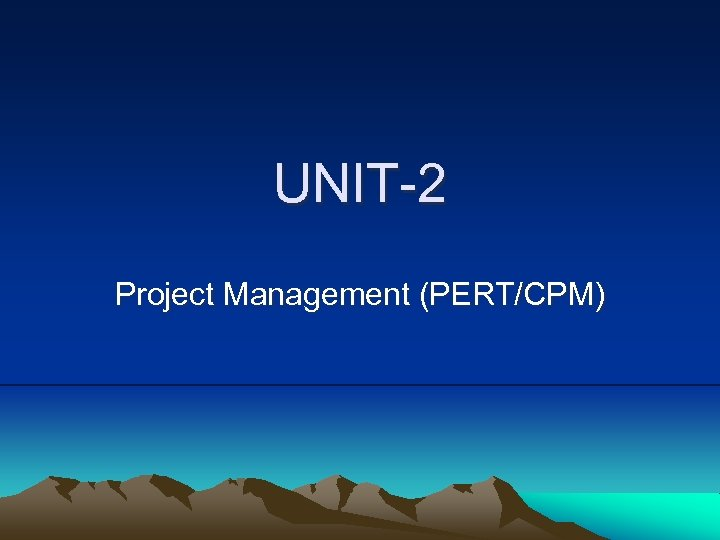UNIT-2 Project Management (PERT/CPM)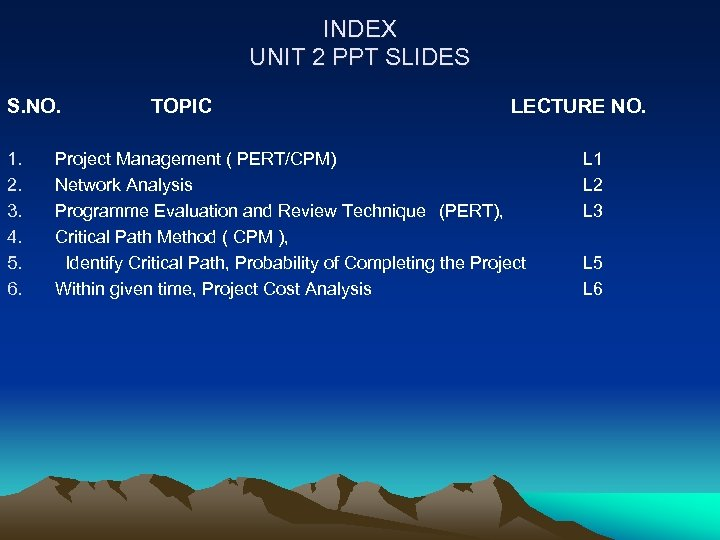INDEX UNIT 2 PPT SLIDES S. NO. 1. 2. 3. 4. 5. 6. TOPIC LECTURE NO. Project Management ( PERT/CPM) Network Analysis Programme Evaluation and Review Technique (PERT), Critical Path Method ( CPM ), Identify Critical Path, Probability of Completing the Project Within given time, Project Cost Analysis L 1 L 2 L 3 L 5 L 6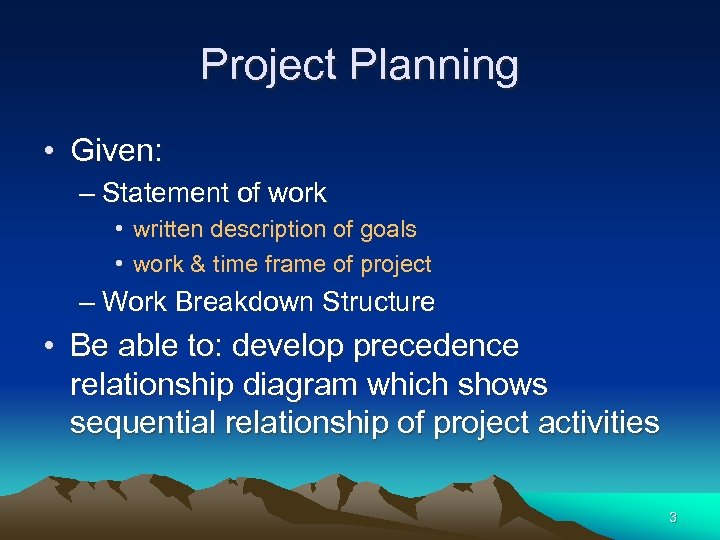Project Planning • Given: – Statement of work • written description of goals • work & time frame of project – Work Breakdown Structure • Be able to: develop precedence relationship diagram which shows sequential relationship of project activities 3Project • “A project is a series of activities directed to accomplishment of a desired objective. ” Plan your work first…. . then work your plan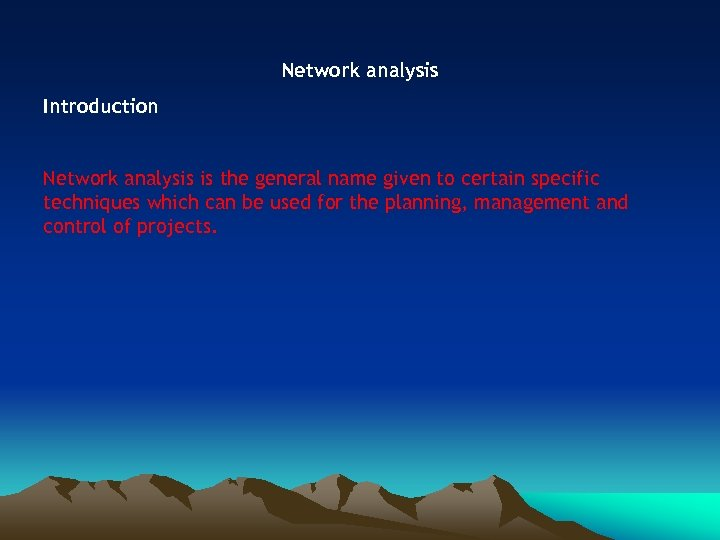Network analysis Introduction Network analysis is the general name given to certain specific techniques which can be used for the planning, management and control of projects.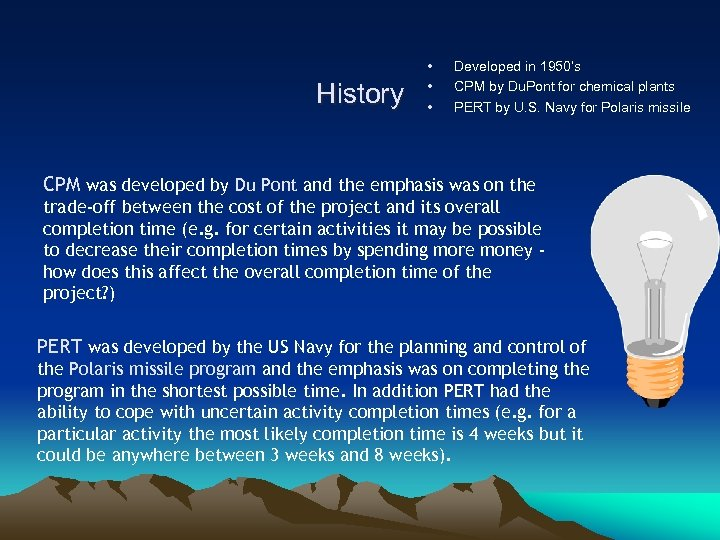History • • • Developed in 1950’s CPM by Du. Pont for chemical plants PERT by U. S. Navy for Polaris missile CPM was developed by Du Pont and the emphasis was on the trade-off between the cost of the project and its overall completion time (e. g. for certain activities it may be possible to decrease their completion times by spending more money how does this affect the overall completion time of the project? ) PERT was developed by the US Navy for the planning and control of the Polaris missile program and the emphasis was on completing the program in the shortest possible time. In addition PERT had the ability to cope with uncertain activity completion times (e. g. for a particular activity the most likely completion time is 4 weeks but it could be anywhere between 3 weeks and 8 weeks).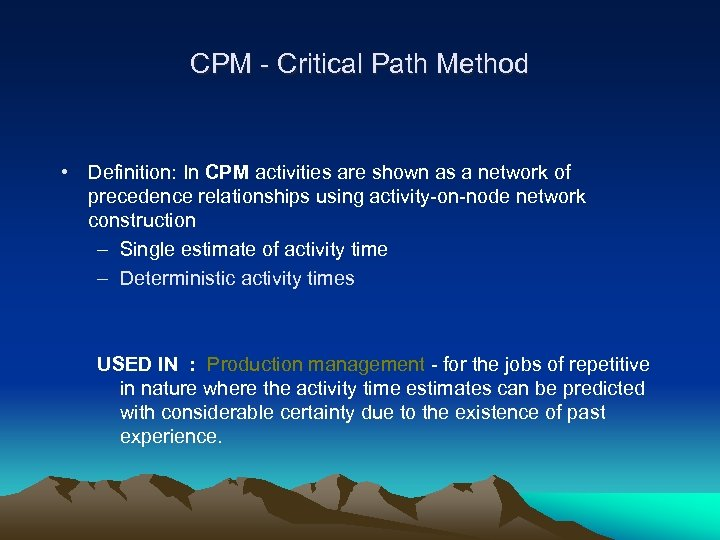CPM - Critical Path Method • Definition: In CPM activities are shown as a network of precedence relationships using activity-on-node network construction – Single estimate of activity time – Deterministic activity times USED IN : Production management - for the jobs of repetitive in nature where the activity time estimates can be predicted with considerable certainty due to the existence of past experience.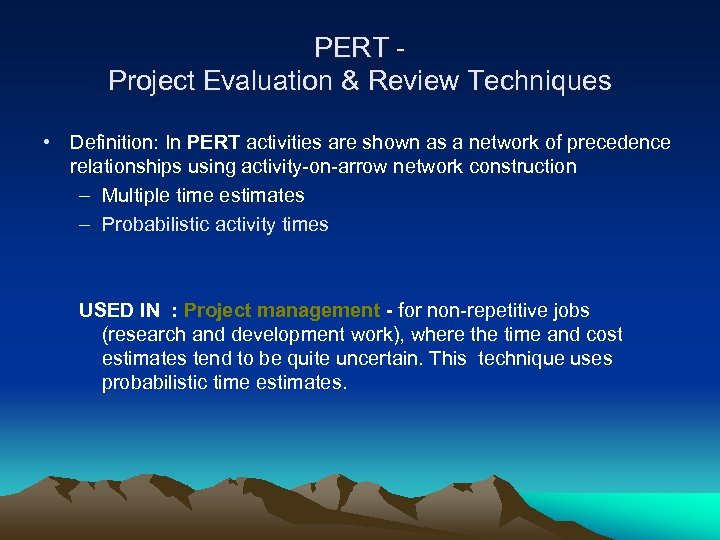PERT Project Evaluation & Review Techniques • Definition: In PERT activities are shown as a network of precedence relationships using activity-on-arrow network construction – Multiple time estimates – Probabilistic activity times USED IN : Project management - for non-repetitive jobs (research and development work), where the time and cost estimates tend to be quite uncertain. This technique uses probabilistic time estimates.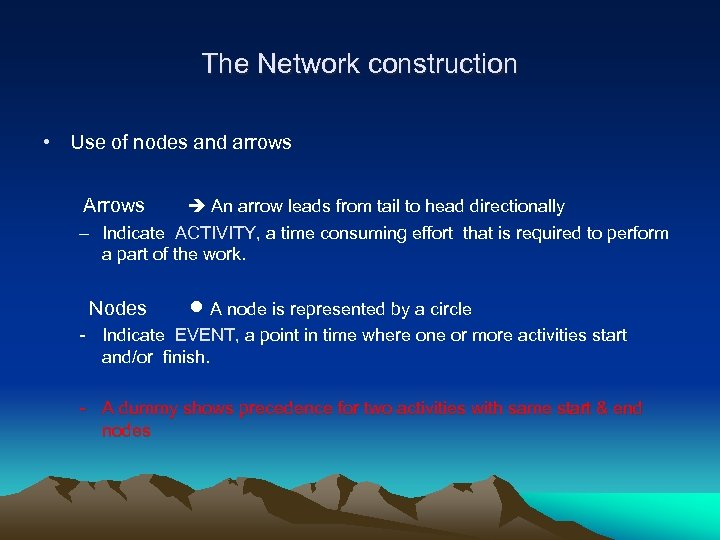The Network construction • Use of nodes and arrows Arrows An arrow leads from tail to head directionally – Indicate ACTIVITY, a time consuming effort that is required to perform a part of the work. Nodes A node is represented by a circle - Indicate EVENT, a point in time where one or more activities start and/or finish. - A dummy shows precedence for two activities with same start & end nodes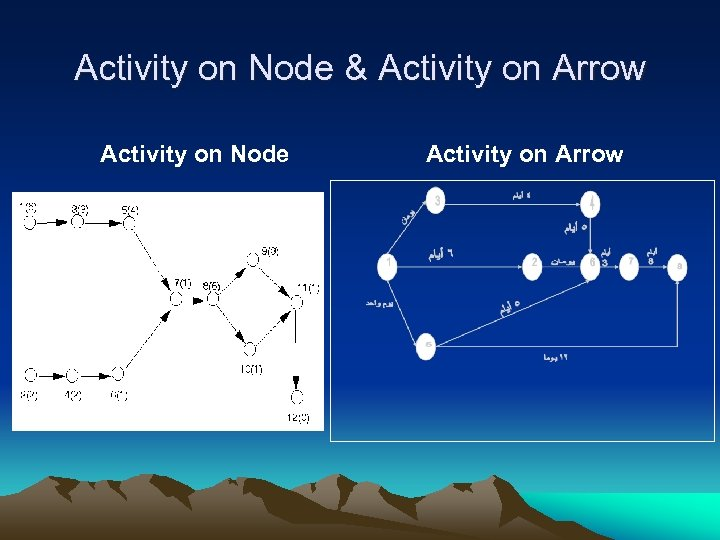Activity on Node & Activity on Arrow Activity on Node Activity on Arrow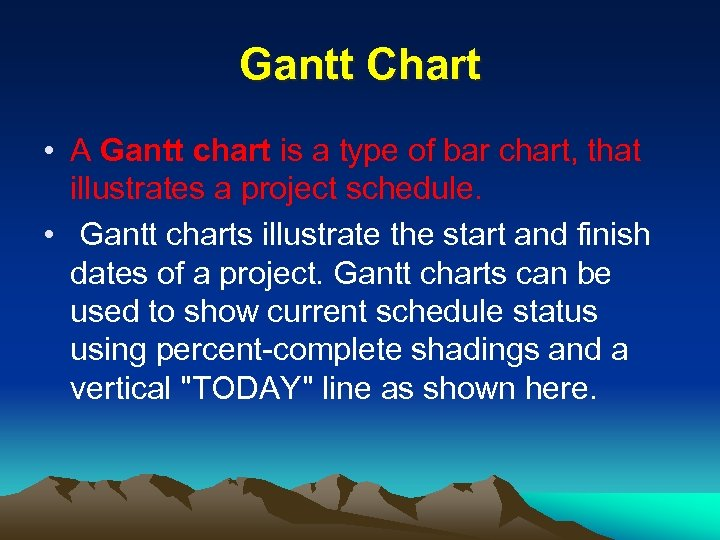Gantt Chart • A Gantt chart is a type of bar chart, that illustrates a project schedule. • Gantt charts illustrate the start and finish dates of a project. Gantt charts can be used to show current schedule status using percent-complete shadings and a vertical "TODAY" line as shown here.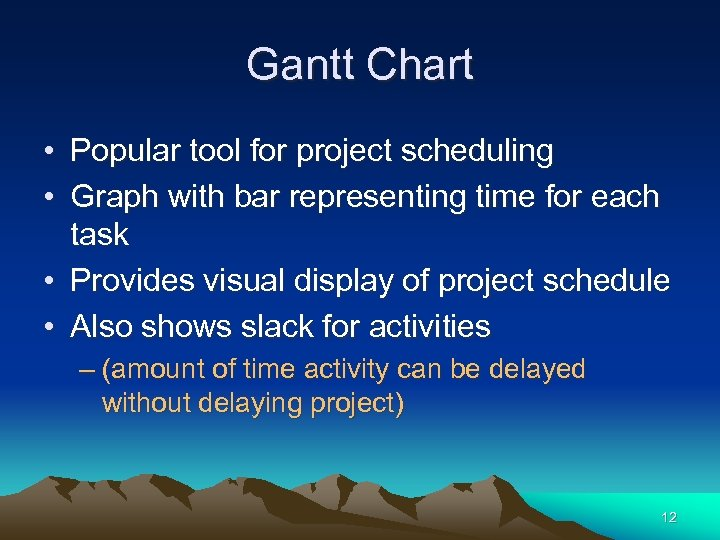Gantt Chart • Popular tool for project scheduling • Graph with bar representing time for each task • Provides visual display of project schedule • Also shows slack for activities – (amount of time activity can be delayed without delaying project) 12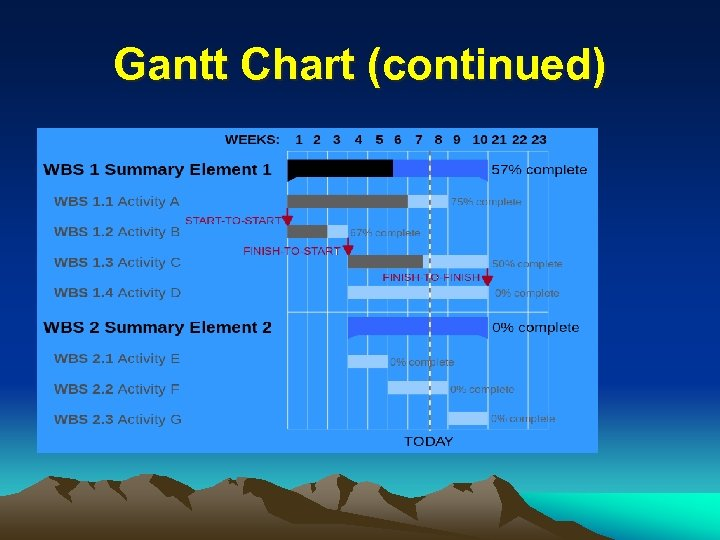Gantt Chart (continued)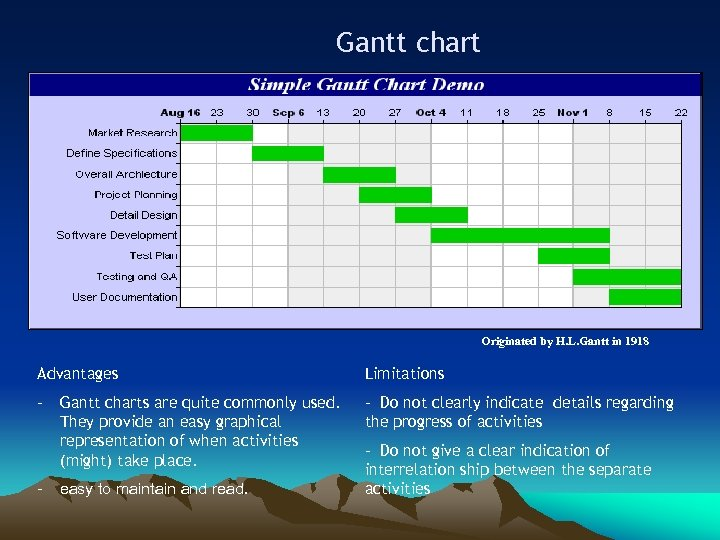Gantt chart Originated by H. L. Gantt in 1918 Advantages Limitations - Gantt charts are quite commonly used. They provide an easy graphical representation of when activities (might) take place. - Do not clearly indicate details regarding the progress of activities - easy to maintain and read. - Do not give a clear indication of interrelation ship between the separate activities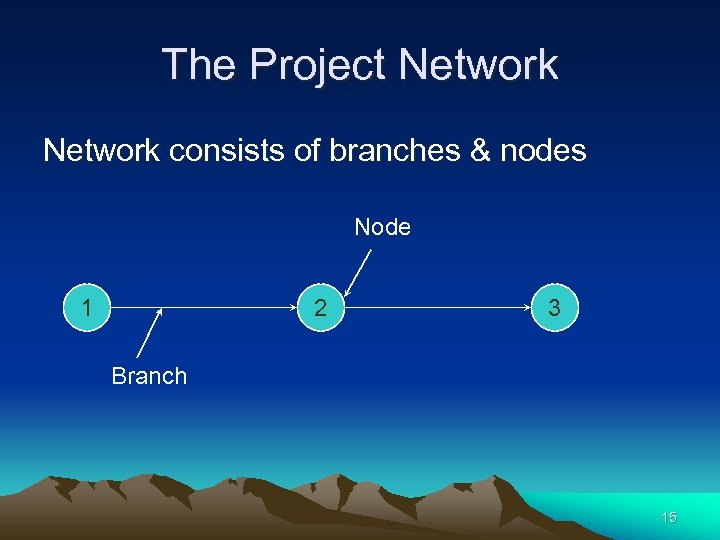The Project Network consists of branches & nodes Node 1 2 3 Branch 15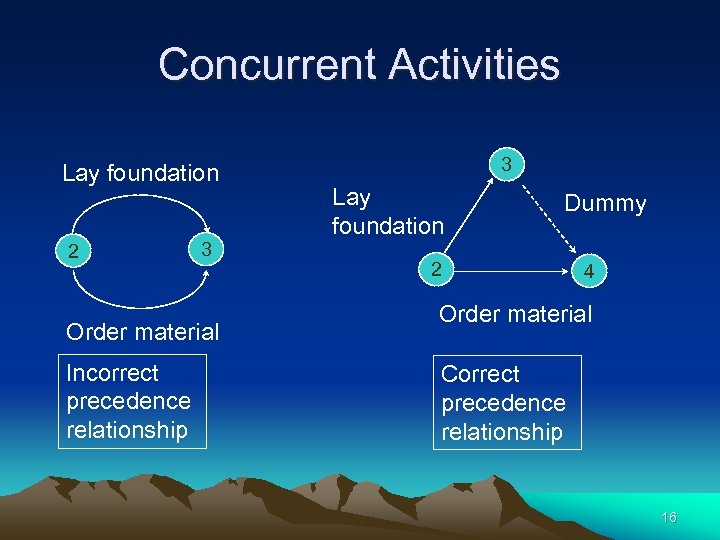Concurrent Activities Lay foundation 2 3 Order material Incorrect precedence relationship 3 Lay foundation Dummy 2 4 Order material Correct precedence relationship 16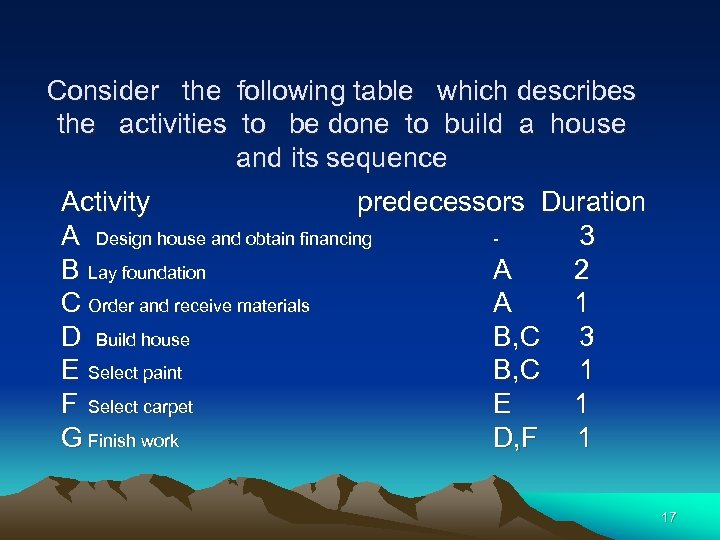Consider the following table which describes the activities to be done to build a house and its sequence Activity predecessors Duration A Design house and obtain financing 3 B Lay foundation A 2 C Order and receive materials A 1 D Build house B, C 3 E Select paint B, C 1 F Select carpet E 1 G Finish work D, F 1 17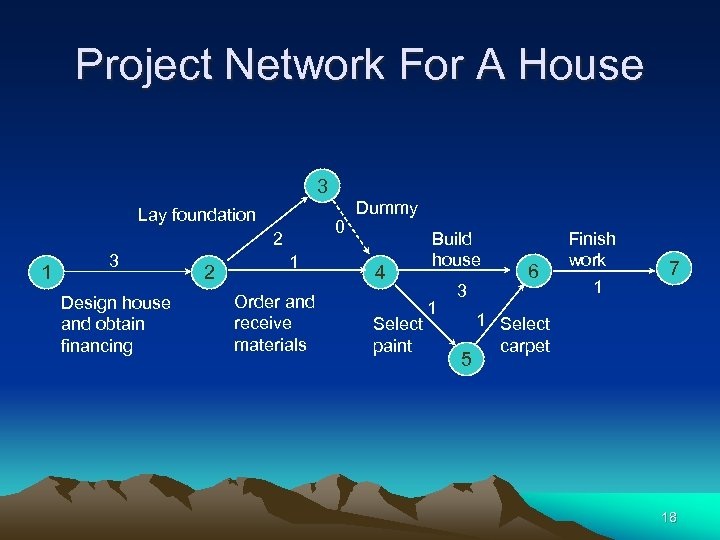Project Network For A House 3 Lay foundation 1 3 Design house and obtain financing 2 2 0 1 Order and receive materials Dummy 4 Select paint Build house 1 3 5 6 Finish work 1 7 1 Select carpet 18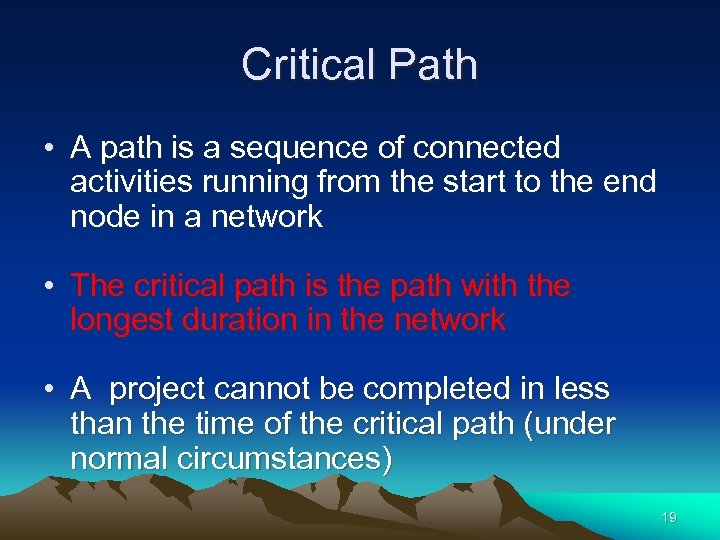Critical Path • A path is a sequence of connected activities running from the start to the end node in a network • The critical path is the path with the longest duration in the network • A project cannot be completed in less than the time of the critical path (under normal circumstances) 19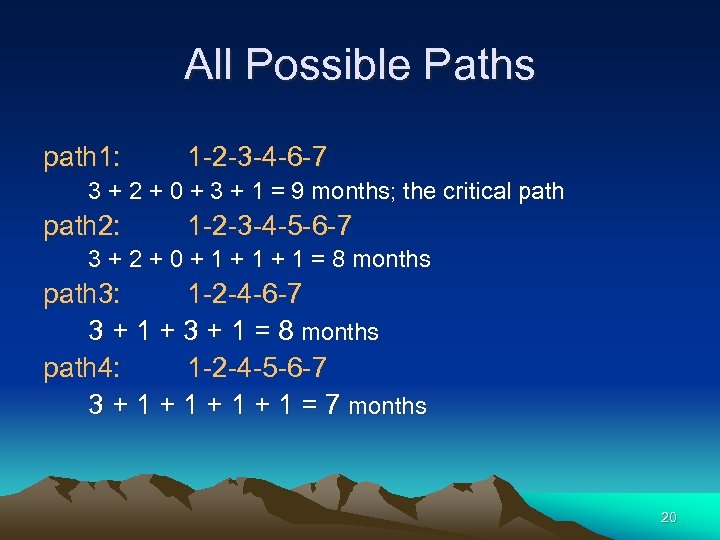All Possible Paths path 1: 1 -2 -3 -4 -6 -7 3 + 2 + 0 + 3 + 1 = 9 months; the critical path 2: 1 -2 -3 -4 -5 -6 -7 3 + 2 + 0 + 1 + 1 = 8 months path 3: 1 -2 -4 -6 -7 3 + 1 + 3 + 1 = 8 months path 4: 1 -2 -4 -5 -6 -7 3 + 1 + 1 = 7 months 20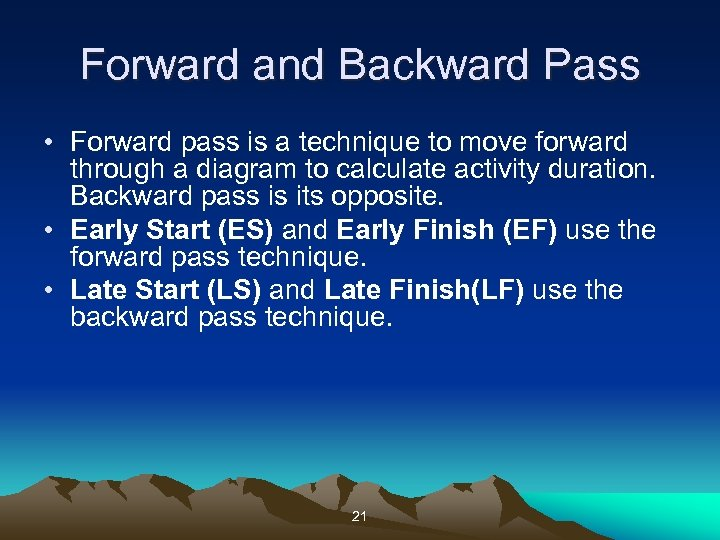Forward and Backward Pass • Forward pass is a technique to move forward through a diagram to calculate activity duration. Backward pass is its opposite. • Early Start (ES) and Early Finish (EF) use the forward pass technique. • Late Start (LS) and Late Finish(LF) use the backward pass technique. 21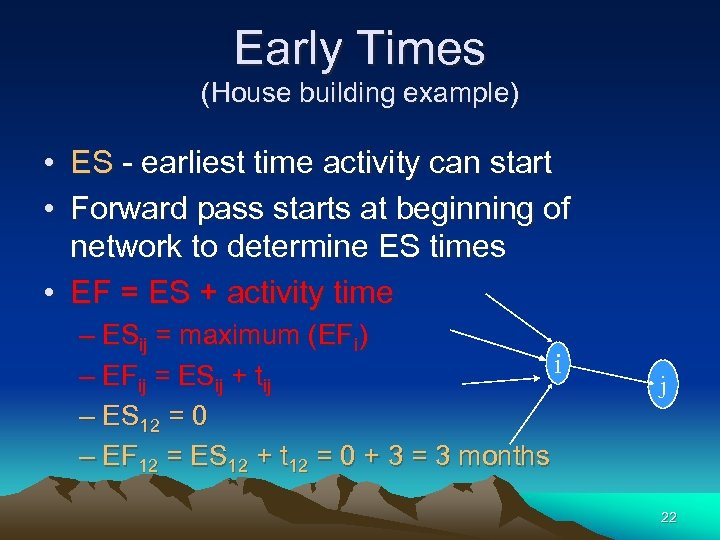Early Times (House building example) • ES - earliest time activity can start • Forward pass starts at beginning of network to determine ES times • EF = ES + activity time – ESij = maximum (EFi) i – EFij = ESij + tij – ES 12 = 0 – EF 12 = ES 12 + t 12 = 0 + 3 = 3 months j 22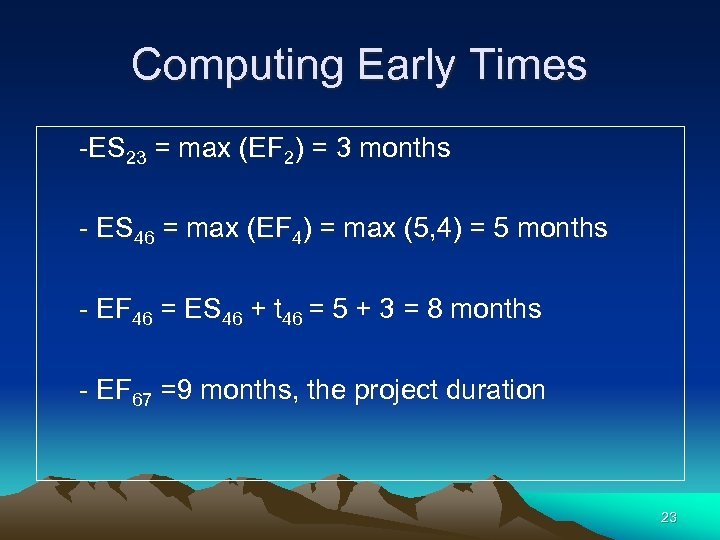Computing Early Times -ES 23 = max (EF 2) = 3 months - ES 46 = max (EF 4) = max (5, 4) = 5 months - EF 46 = ES 46 + t 46 = 5 + 3 = 8 months - EF 67 =9 months, the project duration 23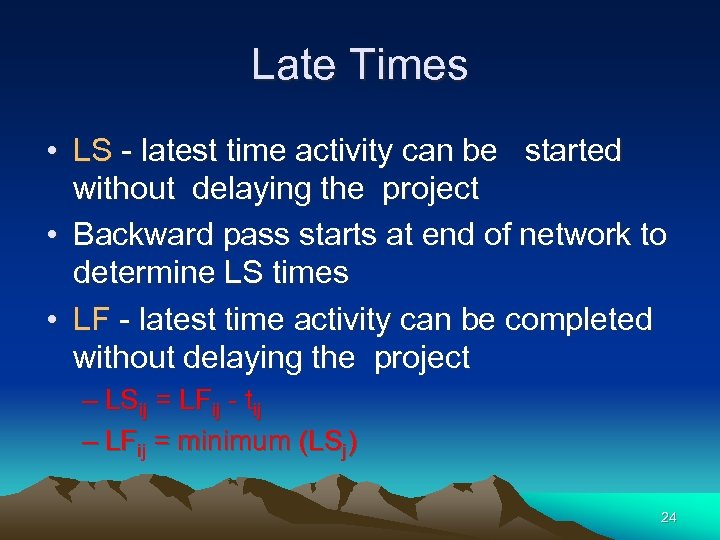Late Times • LS - latest time activity can be started without delaying the project • Backward pass starts at end of network to determine LS times • LF - latest time activity can be completed without delaying the project – LSij = LFij - tij – LFij = minimum (LSj) 24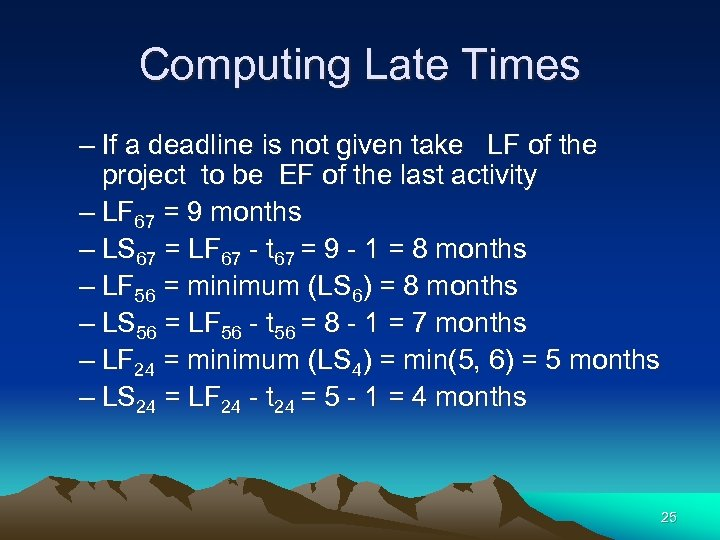Computing Late Times – If a deadline is not given take LF of the project to be EF of the last activity – LF 67 = 9 months – LS 67 = LF 67 - t 67 = 9 - 1 = 8 months – LF 56 = minimum (LS 6) = 8 months – LS 56 = LF 56 - t 56 = 8 - 1 = 7 months – LF 24 = minimum (LS 4) = min(5, 6) = 5 months – LS 24 = LF 24 - t 24 = 5 - 1 = 4 months 25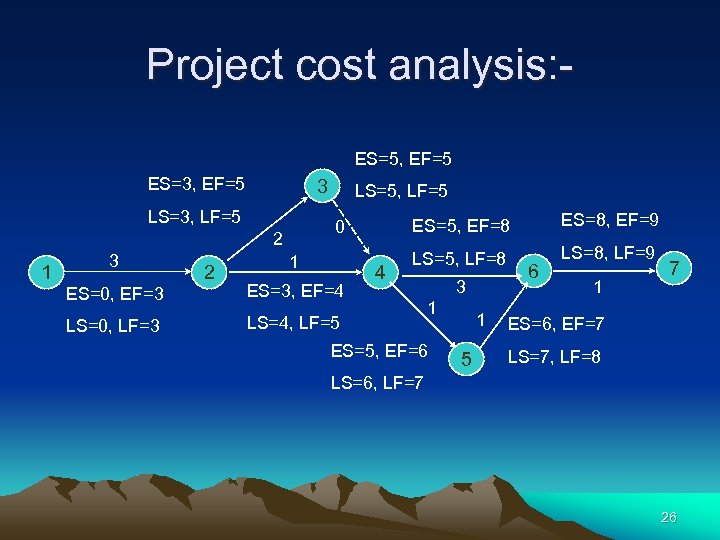Project cost analysis: ES=5, EF=5 ES=3, EF=5 LS=3, LF=5 1 3 ES=0, EF=3 LS=0, LF=3 2 LS=5, LF=5 ES=5, EF=8 0 1 ES=3, EF=4 4 ES=8, EF=9 LS=5, LF=8 LS=8, LF=9 LS=4, LF=5 1 ES=5, EF=6 LS=6, LF=7 3 1 5 6 1 7 ES=6, EF=7 LS=7, LF=8 26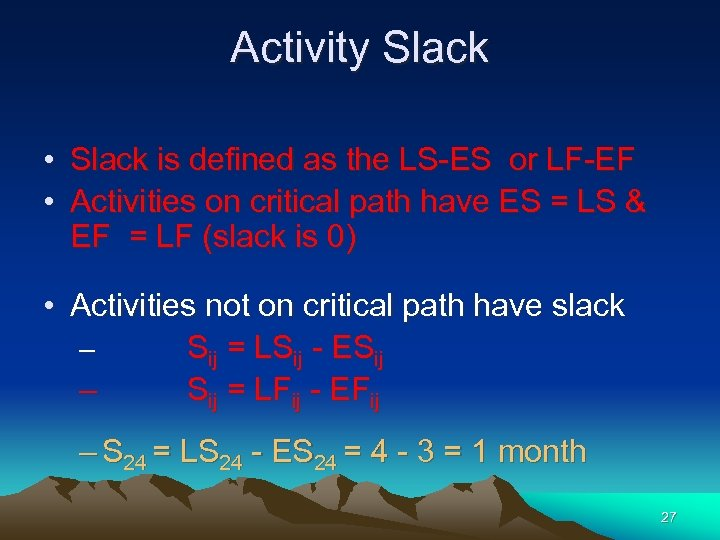Activity Slack • Slack is defined as the LS-ES or LF-EF • Activities on critical path have ES = LS & EF = LF (slack is 0) • Activities not on critical path have slack – Sij = LSij - ESij – Sij = LFij - EFij – S 24 = LS 24 - ES 24 = 4 - 3 = 1 month 27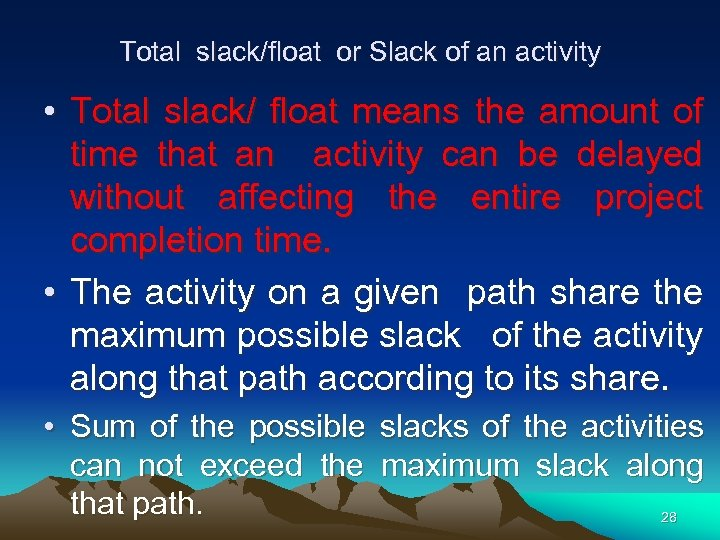Total slack/float or Slack of an activity • Total slack/ float means the amount of time that an activity can be delayed without affecting the entire project completion time. • The activity on a given path share the maximum possible slack of the activity along that path according to its share. • Sum of the possible slacks of the activities can not exceed the maximum slack along that path. 28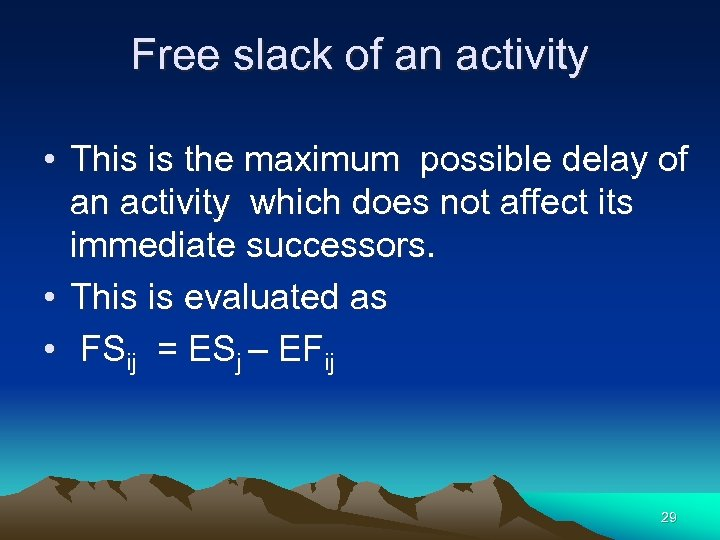Free slack of an activity • This is the maximum possible delay of an activity which does not affect its immediate successors. • This is evaluated as • FSij = ESj – EFij 29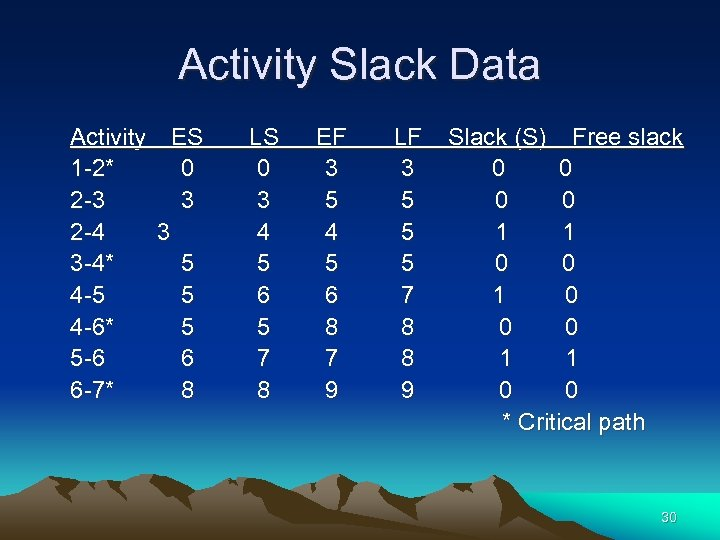Activity Slack Data Activity ES 1 -2* 0 2 -3 3 2 -4 3 3 -4* 5 4 -5 5 4 -6* 5 5 -6 6 6 -7* 8 LS 0 3 4 5 6 5 7 8 EF 3 5 4 5 6 8 7 9 LF 3 5 5 5 7 8 8 9 Slack (S) Free slack 0 0 1 1 0 0 * Critical path 30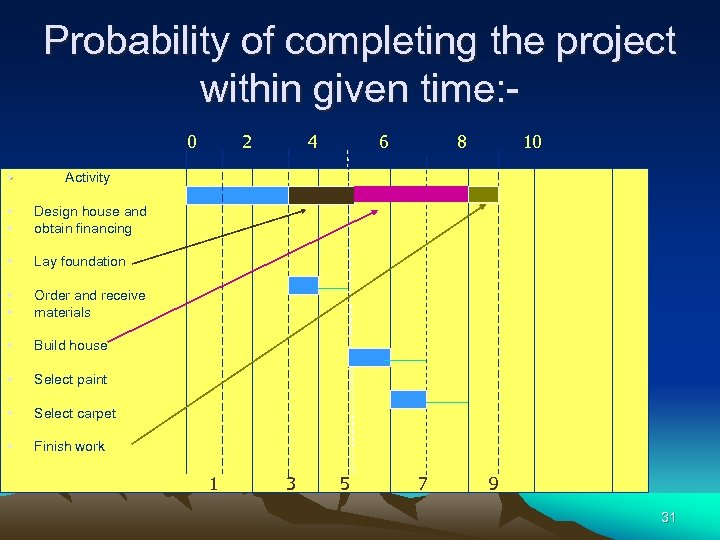Probability of completing the project within given time: 0 • Build house • Select paint • Select carpet • 10 Order and receive materials • 8 Lay foundation • • 6 Design house and obtain financing • 4 Activity • • 2 Finish work 1 3 5 7 9 31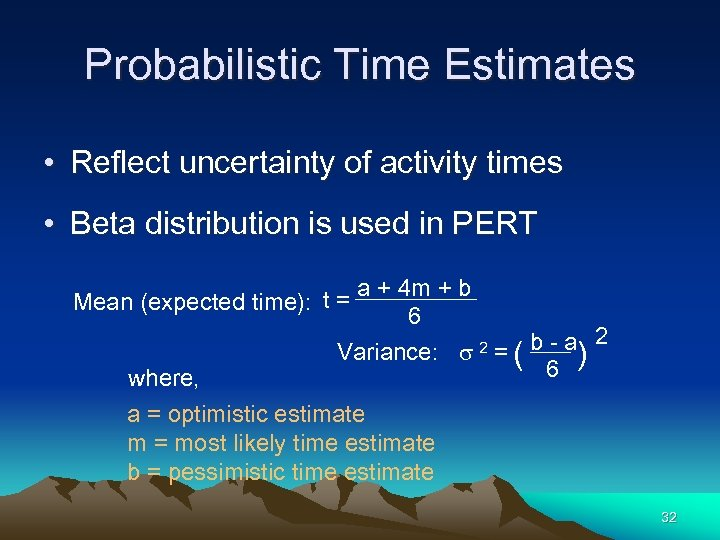Probabilistic Time Estimates • Reflect uncertainty of activity times • Beta distribution is used in PERT a + 4 m + b Mean (expected time): t = 6 where, Variance: s 2 = ( b - a) 6 2 a = optimistic estimate m = most likely time estimate b = pessimistic time estimate 32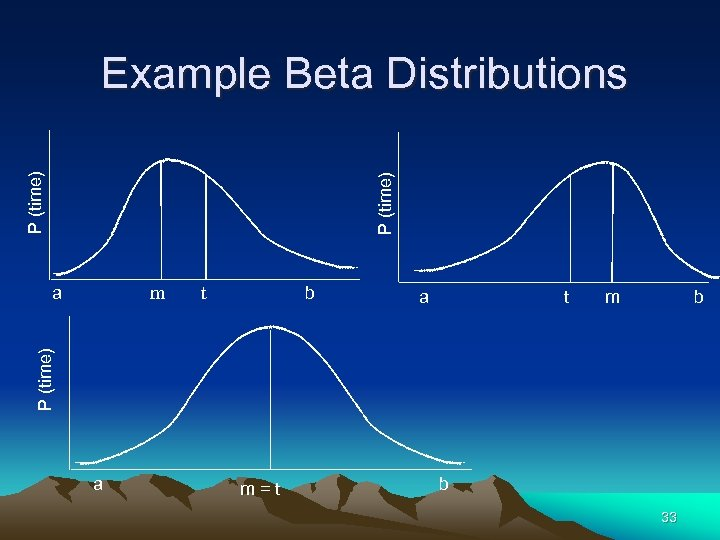P (time) Example Beta Distributions a b t a t m b P (time) m a m=t b 33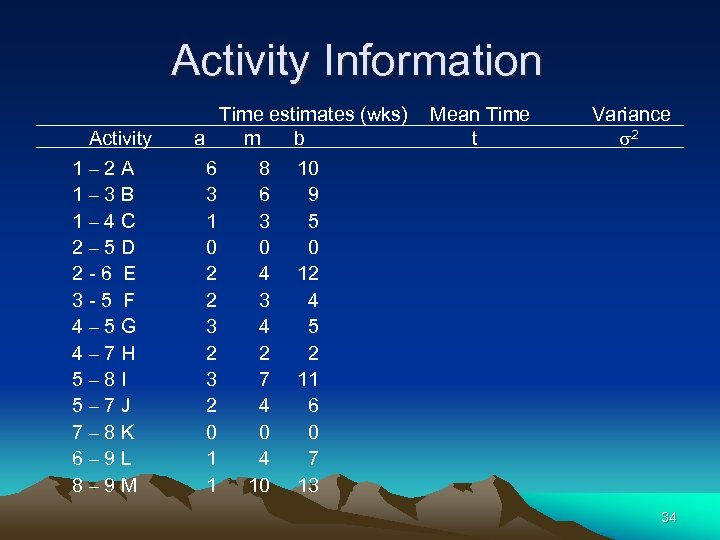Activity Information Activity 1– 2 A 1– 3 B 1– 4 C 2– 5 D 2 -6 E 3 -5 F 4– 5 G 4– 7 H 5– 8 I 5– 7 J 7– 8 K 6– 9 L 8– 9 M Time estimates (wks) a m b 6 3 1 0 2 2 3 2 0 1 1 8 6 3 0 4 3 4 2 7 4 0 4 10 Mean Time t Variance s 2 10 9 5 0 12 4 5 2 11 6 0 7 13 34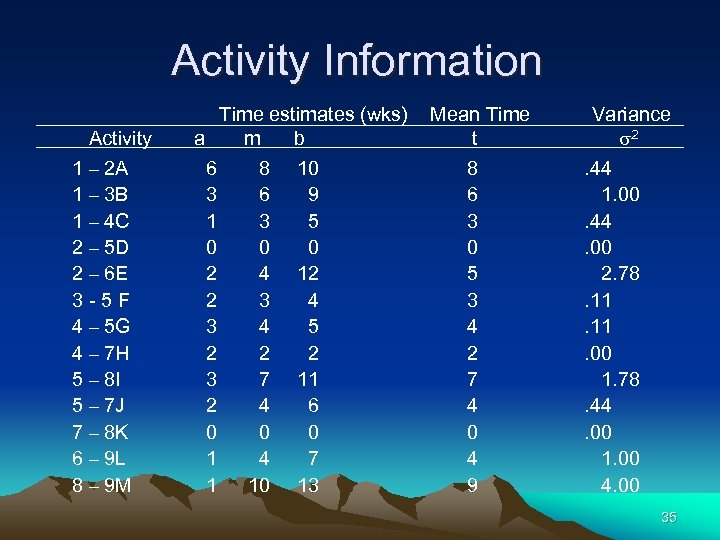Activity Information Activity 1 – 2 A 1 – 3 B 1 – 4 C 2 – 5 D 2 – 6 E 3 -5 F 4 – 5 G 4 – 7 H 5 – 8 I 5 – 7 J 7 – 8 K 6 – 9 L 8 – 9 M Time estimates (wks) a m b 6 3 1 0 2 2 3 2 0 1 1 8 6 3 0 4 3 4 2 7 4 0 4 10 10 9 5 0 12 4 5 2 11 6 0 7 13 Mean Time t 8 6 3 0 5 3 4 2 7 4 0 4 9 Variance s 2. 44 1. 00. 44. 00 2. 78. 11. 00 1. 78. 44. 00 1. 00 4. 00 35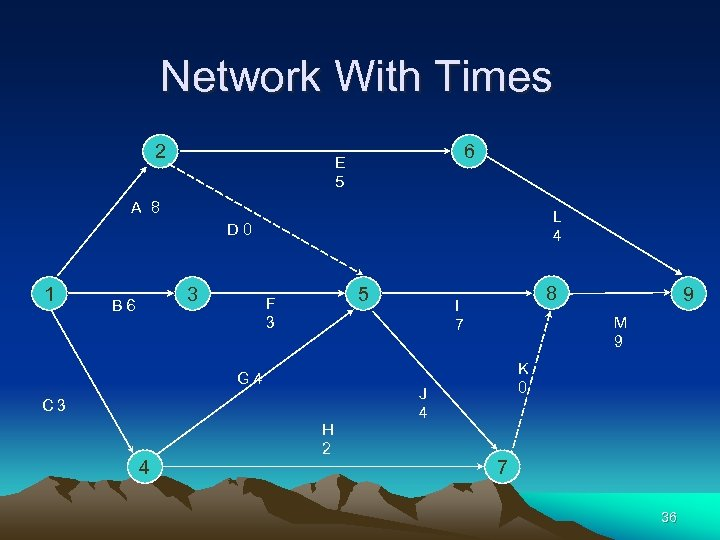Network With Times 2 6 E 5 A 8 L 4 D 0 1 3 B 6 5 F 3 G 4 C 3 4 H 2 8 I 7 9 M 9 K 0 J 4 7 36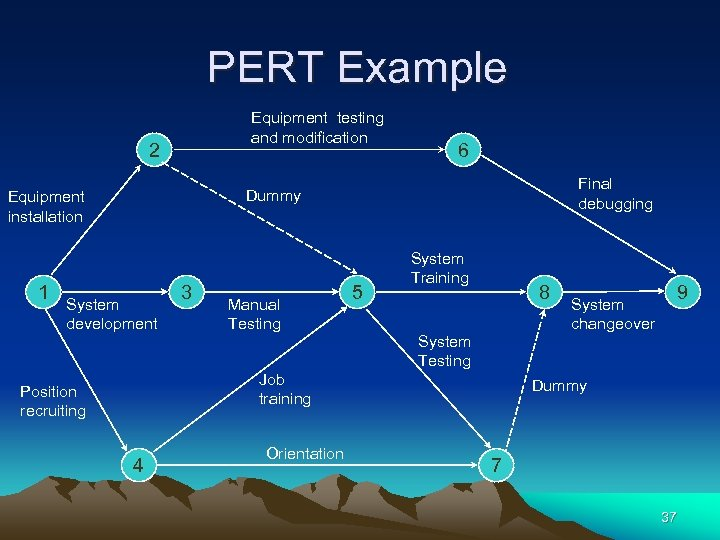PERT Example Equipment testing and modification 2 Final debugging Dummy Equipment installation 1 6 System development 3 Manual Testing Job training Position recruiting 4 Orientation 5 System Training 8 System Testing System changeover 9 Dummy 7 37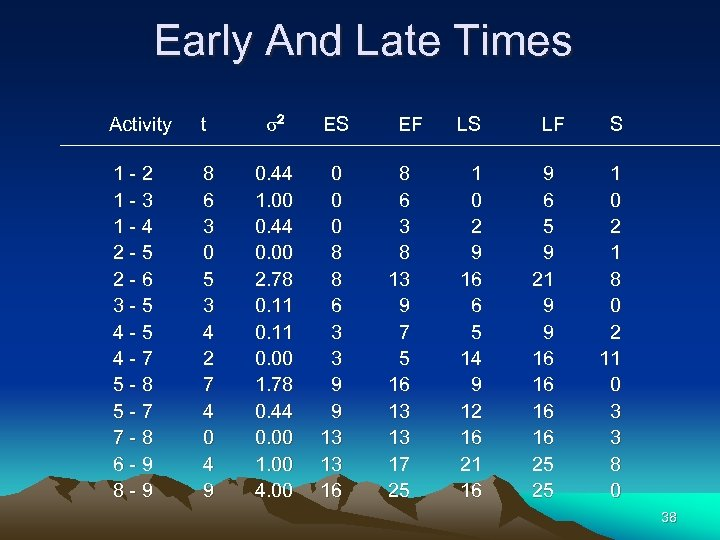Early And Late Times Activity t s 2 ES 1 -2 1 -3 1 -4 2 -5 2 -6 3 -5 4 -7 5 -8 5 -7 7 -8 6 -9 8 6 3 0 5 3 4 2 7 4 0 4 9 0. 44 1. 00 0. 44 0. 00 2. 78 0. 11 0. 00 1. 78 0. 44 0. 00 1. 00 4. 00 0 8 8 6 3 3 9 9 13 13 16 EF 8 6 3 8 13 9 7 5 16 13 13 17 25 LS 1 0 2 9 16 6 5 14 9 12 16 21 16 LF 9 6 5 9 21 9 9 16 16 25 25 S 1 0 2 1 8 0 2 11 0 3 3 8 0 38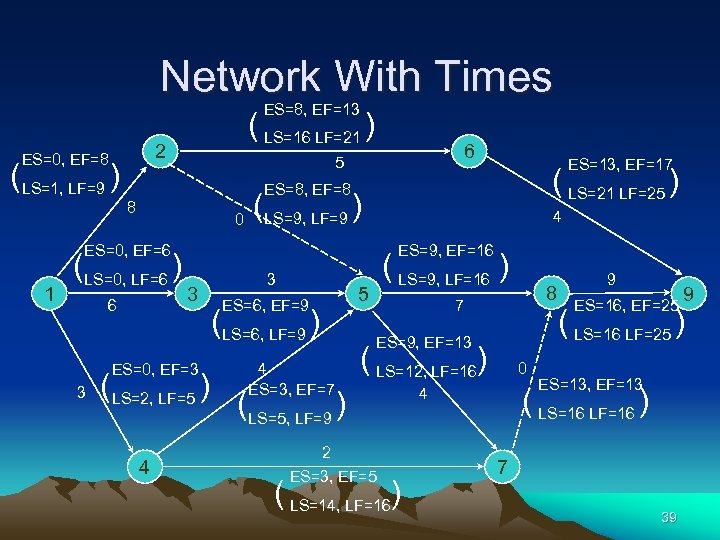Network With Times ES=8, EF=13 2 ES=0, EF=8 (LS=1, LF=9 ) ( LS=16 LF=21 ) 6 5 8 0 (LS=0, LF=6 ) 6 (LS=9, LF=9 ) 4 ES=9, EF=16 3 ES=0, EF=3 3 ( LS=21 LF=25 ) ES=8, EF=8 ES=0, EF=6 1 ES=13, EF=17 (LS=2, LF=5 ) 4 3 ( LS=9, LF=16 ) 5 ES=6, EF=9 (LS=6, LF=9 ) 8 7 ES=9, EF=13 4 ES=3, EF=7 (LS=5, LF=9 ) ( LS=12, LF=16) 2 ES=3, EF=5 ( LS=14, LF=16) 0 9 ES=16, EF=25 ( LS=16 LF=25 ) ES=13, EF=13 ( LS=16 LF=16 ) 4 9 7 39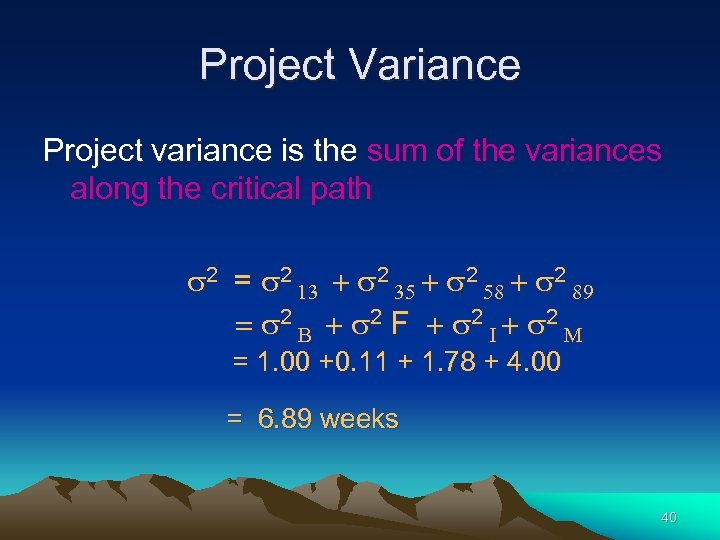Project Variance Project variance is the sum of the variances along the critical path s 2 = s 2 13 + s 2 35 + s 2 58 + s 2 89 = s 2 B + s 2 F + s 2 I + s 2 M = 1. 00 +0. 11 + 1. 78 + 4. 00 = 6. 89 weeks 40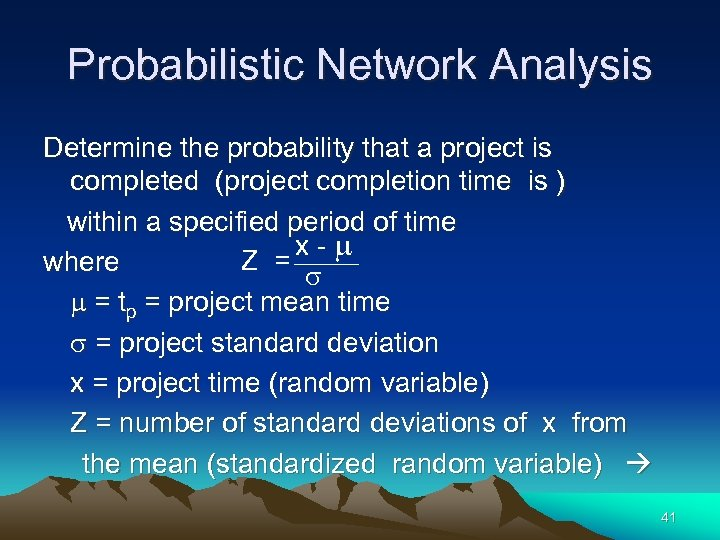Probabilistic Network Analysis Determine the probability that a project is completed (project completion time is ) within a specified period of time x-m Z = where s m = tp = project mean time s = project standard deviation x = project time (random variable) Z = number of standard deviations of x from the mean (standardized random variable) 41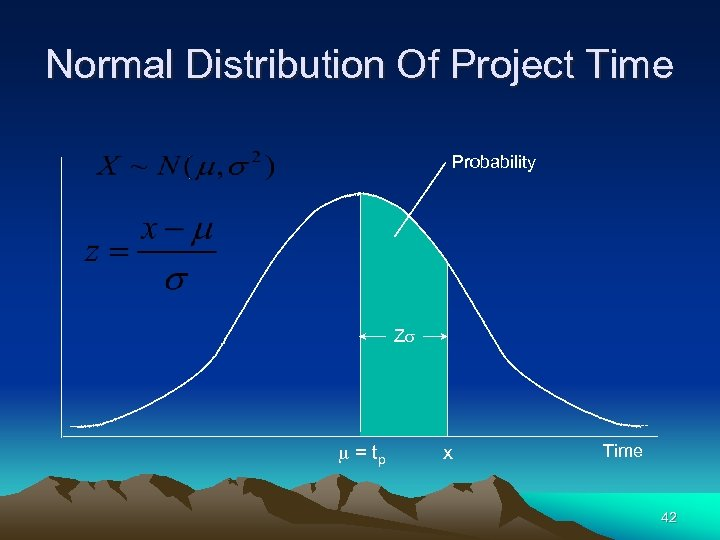Normal Distribution Of Project Time Probability Zs m = tp x Time 42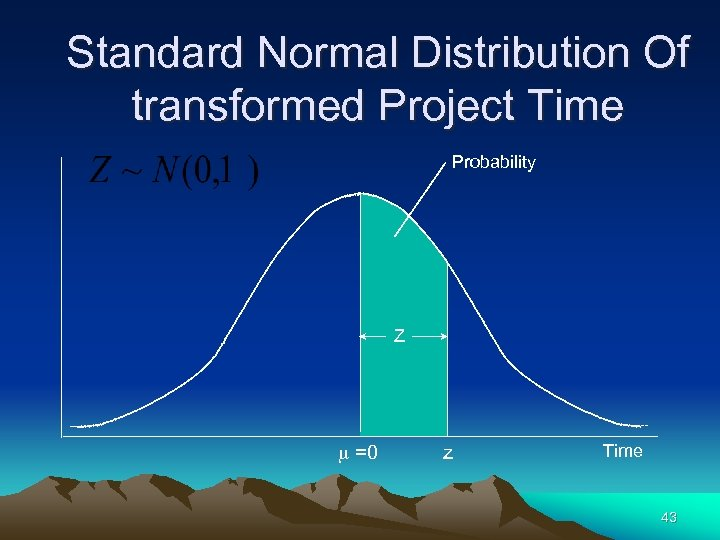Standard Normal Distribution Of transformed Project Time Probability Z m =0 z Time 43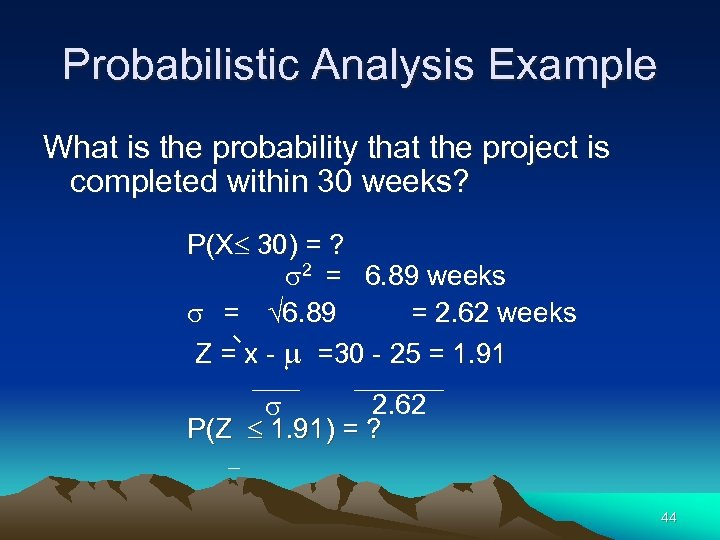Probabilistic Analysis Example What is the probability that the project is completed within 30 weeks? P(X 30) = ? s 2 = 6. 89 weeks s = 6. 89 = 2. 62 weeks Z = x - m =30 - 25 = 1. 91 2. 62 s P(Z 1. 91) = ? 44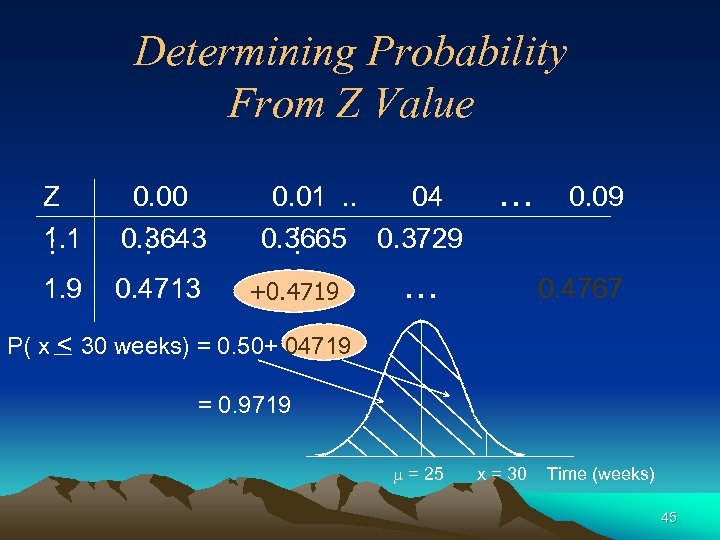Determining Probability From Z Value Z 0. 00 . . 1. 1. . . 0. 3643. 1. 9 0. 4713 0. 01. . 04 . . 0. 3665 0. 3729. +0. 4719 … … 0. 09 0. 4767 P( x < 30 weeks) = 0. 50+ 04719 = 0. 9719 m = 25 x = 30 Time (weeks) 45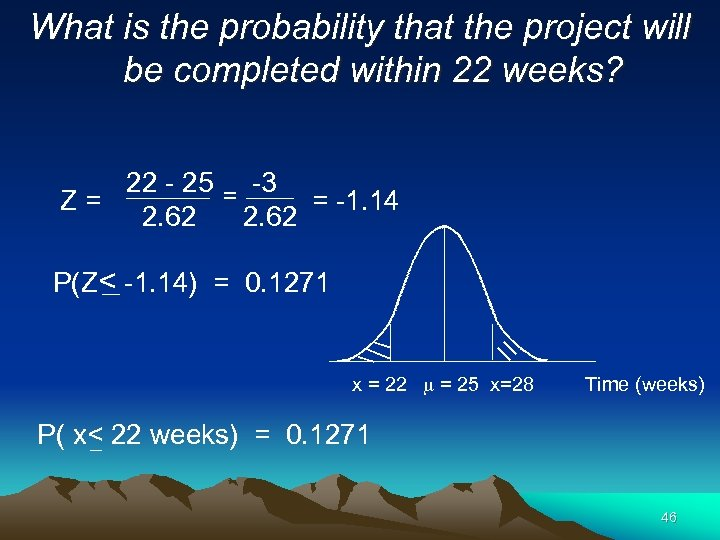What is the probability that the project will be completed within 22 weeks? 22 - 25 = -3 = -1. 14 Z= 2. 62 P(Z< -1. 14) = 0. 1271 x = 22 m = 25 x=28 Time (weeks) P( x< 22 weeks) = 0. 1271 46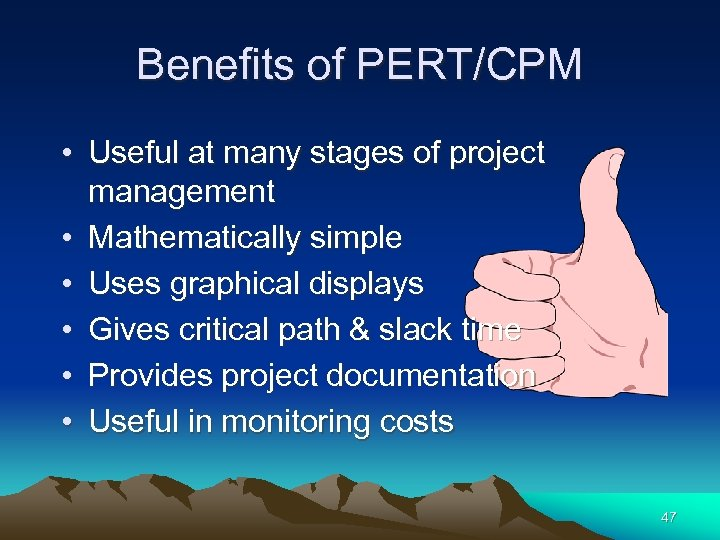Benefits of PERT/CPM • Useful at many stages of project management • Mathematically simple • Uses graphical displays • Gives critical path & slack time • Provides project documentation • Useful in monitoring costs 47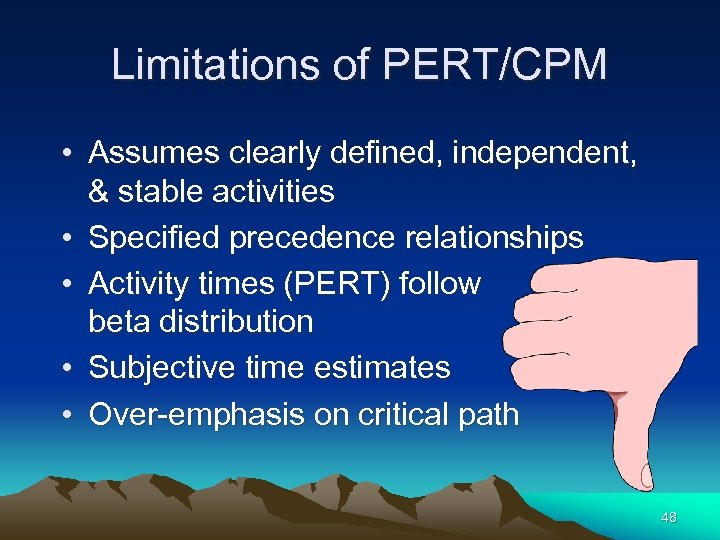Limitations of PERT/CPM • Assumes clearly defined, independent, & stable activities • Specified precedence relationships • Activity times (PERT) follow beta distribution • Subjective time estimates • Over-emphasis on critical path 48